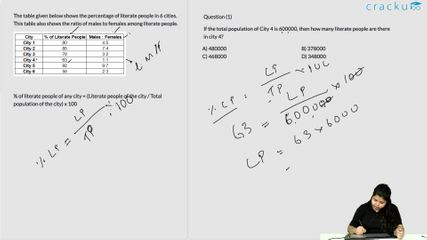### 2017 SSC CGL 09 Aug Shift-2 Question 100

Instructions

The table given below shows the percentage of literate people in 6 cities. This table also shows the ratio of males to females among literate people.

Question 100

# The population of the 6 cities are 250000, 200000, 220000, 300000, 150000 and 400000 respectively. Which is the correct order of the number of literate people in these cities?

Solution

Number of literate people in the cities :

City 1 : $$\frac{80}{100}\times250,000$$ = 200,000

City 2 : $$\frac{85}{100}\times200,000$$ = 170,000

City 3 : $$\frac{78}{100}\times220,000$$ = 171,600

City 4 : $$\frac{63}{100}\times300,000$$ = 189,000

City 5 : $$\frac{92}{100}\times150,000$$ = 138,000

City 6 : $$\frac{58}{100}\times400,000$$ = 232,000

=> City 6 > City 1 > City 4 > City 3 > City 2 > City 5

=> Ans - (D)

### View Video Solution